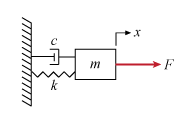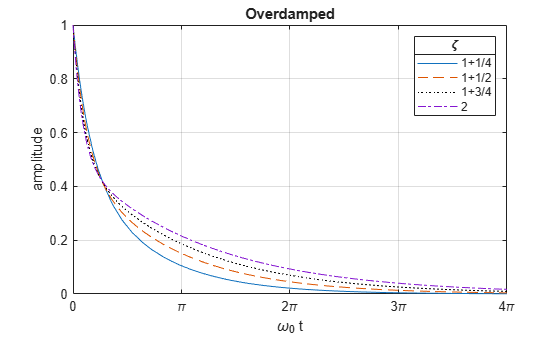Main Content

# The Physics of the Damped Harmonic Oscillator

This example explores the physics of the damped harmonic oscillator by

• solving the equations of motion in the case of no driving forces,

• investigating the cases of under-, over-, and critical-damping### Contents

1. Derive Equation of Motion

2. Solve the Equation of Motion (F = 0)

3. Underdamped Case ($\zeta <1$)

4. Overdamped Case ($\zeta >1$)

5. Critically Damped Case ($\zeta =1$)

6. Conclusion

### 1. Derive Equation of Motion

Consider a forced harmonic oscillator with damping shown below. Model the resistance force as proportional to the speed with which the oscillator moves.Define the equation of motion where

• $m$ is the mass

• $c$ is the damping coefficient

• $k$ is the spring constant

• $F$ is a driving force

syms x(t) m c k F(t) eq = m*diff(x,t,t) + c*diff(x,t) + k*x == F
eq(t) =  

Rewrite the equation using $\mathit{c}=\mathit{m}\text{\hspace{0.17em}}\gamma$ and $\mathit{k}=\mathit{m}\text{\hspace{0.17em}}{\omega }_{0}^{2}$.

syms gamma omega_0 eq = subs(eq, [c k], [m*gamma, m*omega_0^2])
eq(t) =  

Divide out the mass $\mathit{m}$. Now we have the equation in a convenient form to analyze.

eq = collect(eq, m)/m
eq(t) =  

### 2. Solve the Equation of Motion where F = 0

Solve the equation of motion using dsolve in the case of no external forces where $F=0$. Use the initial conditions of unit displacement and zero velocity.

vel = diff(x,t); cond = [x(0) == 1, vel(0) == 0]; eq = subs(eq,F,0); sol = dsolve(eq, cond)
sol =  

Examine how to simplify the solution by expanding it.

sol = expand(sol)
sol =  

Notice that each term has a factor of ${\sigma }_{1}$, or ${e}^{-\gamma t/2}$, use collect to gather these terms

sol = collect(sol, exp(-gamma*t/2))
sol =  

The term $\sqrt{{\gamma }^{2}-4{\omega }_{0}^{2}}$ appears in various parts of the solution. Rewrite it in a simpler form by introducing the damping ratio $\zeta \equiv \frac{\gamma }{2{\omega }_{0}}$.

Substituting ζ into the term above gives:

$\sqrt{{\gamma }^{2}-4{\omega }_{0}^{2}}=2{\omega }_{0}\sqrt{{\left(\frac{\gamma }{2{\omega }_{0}}\right)}^{2}-1}=2{\omega }_{0}\sqrt{{\zeta }^{2}-1},$

syms zeta; sol = subs(sol, ... sqrt(gamma^2 - 4*omega_0^2), ... 2*omega_0*sqrt(zeta^2-1))
sol =  

Further simplify the solution by substituting $\gamma$ in terms of ${\omega }_{0}$ and $\zeta$,

sol = subs(sol, gamma, 2*zeta*omega_0)
sol =  

We have derived the general solution for the motion of the damped harmonic oscillator with no driving forces. Next, we'll explore three special cases of the damping ratio $\zeta$ where the motion takes on simpler forms. These cases are called

• underdamped $\left(\zeta <1\right)$,

• overdamped $\left(\zeta >1\right)$, and

• critically damped $\left(\zeta =1\right)$.

### 3. Underdamped Case ($\zeta <1$)

If $\zeta <1$, then $\sqrt{{\zeta }^{2}-1}=i\sqrt{1-{\zeta }^{2}}$ is purely imaginary

solUnder = subs(sol, sqrt(zeta^2-1), 1i*sqrt(1-zeta^2))
solUnder =  

Notice the terms ${\mathit{e}}^{\mathit{i}\text{\hspace{0.17em}}{\omega }_{0}\text{\hspace{0.17em}}\mathit{t}\sqrt{{\zeta }^{2}-1}}±\text{\hspace{0.17em}}{\mathit{e}}^{-{\mathit{i}\text{\hspace{0.17em}}\omega }_{0}\text{\hspace{0.17em}}\mathit{t}\sqrt{{\zeta }^{2}-1}}$ in the above equation and recall the identity ${\mathit{e}}^{\mathit{i}\text{\hspace{0.17em}}\mathit{x}}=\mathrm{cos}\left(\mathit{x}\right)+\mathit{i}\text{\hspace{0.17em}}\mathrm{sin}\left(\mathit{x}\right).$

Rewrite the solution in terms of $\mathrm{cos}$.

solUnder = coeffs(solUnder, zeta); solUnder = solUnder(1); c = exp(-omega_0 * zeta * t); solUnder = c * rewrite(solUnder / c, 'cos')
solUnder = ${\mathrm{e}}^{-{\omega }_{0} t \zeta } \mathrm{cos}\left({\omega }_{0} t \sqrt{1-{\zeta }^{2}}\right)$
solUnder(t, omega_0, zeta) = solUnder
solUnder(t, omega_0, zeta) = ${\mathrm{e}}^{-{\omega }_{0} t \zeta } \mathrm{cos}\left({\omega }_{0} t \sqrt{1-{\zeta }^{2}}\right)$

The system oscillates at a natural frequency of ${\omega }_{0}\sqrt{1-{\zeta }^{2}}$ and decays at an exponential rate of $1/{\omega }_{0}\phantom{\rule{0.16666666666666666em}{0ex}}\zeta$.

Plot the solution with fplot as a function of ${\omega }_{0}t$ and $\zeta$.

z = [0 1/4 1/2 3/4]; w = 1; T = 4*pi; lineStyle = {'-','--',':k','-.'}; fplot(@(t)solUnder(t, w, z(1)), [0 T], lineStyle{1}); hold on; for k = 2:numel(z) fplot(@(t)solUnder(t, w, z(k)), [0 T], lineStyle{k}); end hold off; grid on; xticks(T*linspace(0,1,5)); xticklabels({'0','\pi','2\pi','3\pi','4\pi'}); xlabel('t / \omega_0'); ylabel('amplitude'); lgd = legend('0','1/4','1/2','3/4'); title(lgd,'\zeta'); title('Underdamped');### 4. Overdamped Case ($\zeta >1$)

If $\zeta >1$, then $\sqrt{{\zeta }^{2}-1}$ is purely real and the solution can be rewritten as

solOver = sol
solOver =  
solOver = coeffs(solOver, zeta); solOver = solOver(1)
solOver =  ${\mathrm{e}}^{-{\omega }_{0} t \zeta } \left(\frac{{\mathrm{e}}^{{\omega }_{0} t \sqrt{{\zeta }^{2}-1}}}{2}+\frac{{\mathrm{e}}^{-{\omega }_{0} t \sqrt{{\zeta }^{2}-1}}}{2}\right)$

Notice the terms $\frac{\left({\mathit{e}}^{{\omega }_{0}\mathit{t}\sqrt{{\zeta }^{2}-1}}+{\mathit{e}}^{-{\omega }_{0}\mathit{t}\sqrt{{\zeta }^{2}-1}}\right)}{2}$ and recall the identity $\mathrm{cosh}\left(\mathit{x}\right)=\frac{{\mathit{e}}^{\mathit{x}}+{\mathit{e}}^{-\mathit{x}}}{2}$.

Rewrite the expression in terms of $\mathrm{cosh}$.

c = exp(-omega_0*t*zeta); solOver = c*rewrite(solOver / c, 'cosh')
solOver = $\mathrm{cosh}\left({\omega }_{0} t \sqrt{{\zeta }^{2}-1}\right) {\mathrm{e}}^{-{\omega }_{0} t \zeta }$
solOver(t, omega_0, zeta) = solOver
solOver(t, omega_0, zeta) = $\mathrm{cosh}\left({\omega }_{0} t \sqrt{{\zeta }^{2}-1}\right) {\mathrm{e}}^{-{\omega }_{0} t \zeta }$

Plot the solution to see that it decays without oscillating.

z = 1 + [1/4 1/2 3/4 1]; w = 1; T = 4*pi; lineStyle = {'-','--',':k','-.'}; fplot(@(t)solOver(t, w, z(1)), [0 T], lineStyle{1}); hold on; for k = 2:numel(z) fplot(@(t)solOver(t, w, z(k)), [0 T], lineStyle{k}); end hold off; grid on; xticks(T*linspace(0,1,5)); xticklabels({'0','\pi','2\pi','3\pi','4\pi'}); xlabel('\omega_0 t'); ylabel('amplitude'); lgd = legend('1+1/4','1+1/2','1+3/4','2'); title(lgd,'\zeta'); title('Overdamped');### 5. Critically Damped Case ($\zeta =1$)

If $\zeta =1$, then the solution simplifies to

solCritical(t, omega_0) = limit(sol, zeta, 1)
solCritical(t, omega_0) = ${\mathrm{e}}^{-{\omega }_{0} t} \left({\omega }_{0} t+1\right)$

Plot the solution for the critically damped case.

w = 1; T = 4*pi; fplot(solCritical(t, w), [0 T]) xlabel('\omega_0 t'); ylabel('x'); title('Critically damped, \zeta = 1'); grid on; xticks(T*linspace(0,1,5)); xticklabels({'0','\pi','2\pi','3\pi','4\pi'});### 6. Conclusion

We have examined the different damping states for the harmonic oscillator by solving the ODEs which represents its motion using the damping ratio $\zeta$. Plot all three cases together to compare and contrast them.

zOver = pi; zUnder = 1/zOver; w = 1; T = 2*pi; lineStyle = {'-','--',':k'}; fplot(@(t)solOver(t, w, zOver), [0 T], lineStyle{1},'LineWidth',2); hold on; fplot(solCritical(t, w), [0 T], lineStyle{2},'LineWidth',2) fplot(@(t)solUnder(t, w, zUnder), [0 T], lineStyle{3},'LineWidth',2); hold off; textColor = lines(3); text(3*pi/2, 0.3 , 'over-damped' ,'Color',textColor(1,:)); text(pi*3/4, 0.05, 'critically-damped','Color',textColor(2,:)); text(pi/8 , -0.1, 'under-damped'); grid on; xlabel('\omega_0 t'); ylabel('amplitude'); xticks(T*linspace(0,1,5)); xticklabels({'0','\pi/2','\pi','3\pi/2','2\pi'}); yticks((1/exp(1))*[-1 0 1 2 exp(1)]); yticklabels({'-1/e','0','1/e','2/e','1'}); lgd = legend('\pi','1','1/\pi'); title(lgd,'\zeta'); title('Damped Harmonic Oscillator');## Support

#### Mathematical Modeling with Symbolic Math Toolbox

Get examples and videos